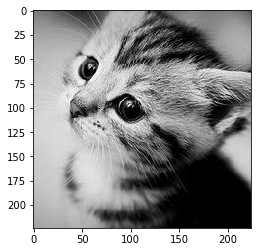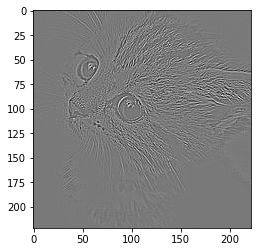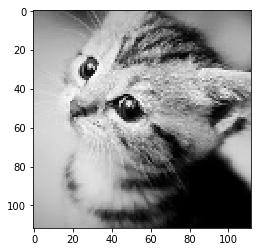# 卷积模块介绍

## 卷积

``````import numpy as np
import torch
from torch import nn
import torch.nn.functional as F
from PIL import Image
import matplotlib.pyplot as plt
%matplotlib inline
``````
``````im = Image.open('./cat.png').convert('L') # 读入一张灰度图的图片
im = np.array(im, dtype='float32') # 将其转换为一个矩阵
``````
``````# 可视化图片
plt.imshow(im.astype('uint8'), cmap='gray')
``````
``````<matplotlib.image.AxesImage at 0x108634c88>
````````````# 将图片矩阵转化为 pytorch tensor，并适配卷积输入的要求
im = torch.from_numpy(im.reshape((1, 1, im.shape, im.shape)))
``````

``````# 使用 nn.Conv2d
conv1 = nn.Conv2d(1, 1, 3, bias=False) # 定义卷积

sobel_kernel = np.array([[-1, -1, -1], [-1, 8, -1], [-1, -1, -1]], dtype='float32') # 定义轮廓检测算子
sobel_kernel = sobel_kernel.reshape((1, 1, 3, 3)) # 适配卷积的输入输出
conv1.weight.data = torch.from_numpy(sobel_kernel) # 给卷积的 kernel 赋值

edge1 = conv1(Variable(im)) # 作用在图片上
edge1 = edge1.data.squeeze().numpy() # 将输出转换为图片的格式
``````

``````plt.imshow(edge1, cmap='gray')
``````
``````<matplotlib.image.AxesImage at 0x109a4a128>
````````````# 使用 F.conv2d
sobel_kernel = np.array([[-1, -1, -1], [-1, 8, -1], [-1, -1, -1]], dtype='float32') # 定义轮廓检测算子
sobel_kernel = sobel_kernel.reshape((1, 1, 3, 3)) # 适配卷积的输入输出
weight = Variable(torch.from_numpy(sobel_kernel))

edge2 = F.conv2d(Variable(im), weight) # 作用在图片上
edge2 = edge2.data.squeeze().numpy() # 将输出转换为图片的格式
plt.imshow(edge2, cmap='gray')
``````
``````<matplotlib.image.AxesImage at 0x10a103eb8>
``````## 池化层

``````# 使用 nn.MaxPool2d
pool1 = nn.MaxPool2d(2, 2)
print('before max pool, image shape: {} x {}'.format(im.shape, im.shape))
small_im1 = pool1(Variable(im))
small_im1 = small_im1.data.squeeze().numpy()
print('after max pool, image shape: {} x {} '.format(small_im1.shape, small_im1.shape))
``````
``````before max pool, image shape: 224 x 224
after max pool, image shape: 112 x 112
``````

``````plt.imshow(small_im1, cmap='gray')
``````
``````<matplotlib.image.AxesImage at 0x10a5867f0>
````````````# F.max_pool2d
print('before max pool, image shape: {} x {}'.format(im.shape, im.shape))
small_im2 = F.max_pool2d(Variable(im), 2, 2)
small_im2 = small_im2.data.squeeze().numpy()
print('after max pool, image shape: {} x {} '.format(small_im1.shape, small_im1.shape))
plt.imshow(small_im2, cmap='gray')
``````
``````before max pool, image shape: 224 x 224
after max pool, image shape: 112 x 112

<matplotlib.image.AxesImage at 0x10a6ac898>
``````